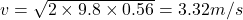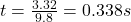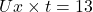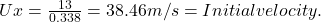## A tennis player serves a tennis ball such that it is moving horizontally when it leaves the racquet. When the ball travels a horizontal dist

Question

A tennis player serves a tennis ball such that it is moving horizontally when it leaves the racquet. When the ball travels a horizontal distance of 13 m, it has dropped 56 cm from its original height when it left the racquet. What was the initial speed, in m/s, of the tennis ball

in progress 0
4 months 2021-09-05T09:56:24+00:00 1 Answers 4 views 0

The initial velocity is 38.46 m/s.

Explanation:

The horizontal distance travel by the tennis ball = 13 m

The height at which the tennis ball dropped = 56 cm

Now calculate the initial speed of tennis ball.

The vertical velocity is zero.

Below is the calculation. Here, first convert centimetre into kilometre. So, height at which ball dropped is 0.56 km.